Select Page

# CBSE Maths 12 Science Application of Derivatives Solutions for MCQ in English

CBSE Maths 12 Science Application of Derivatives Solutions for MCQ in English to enable students to get Solutions in a narrative video format for the specific question.

Expert Teacher provides CBSE Application of Derivatives Maths 12 Science Solutions for MCQ through Video Solutions in English language. This video solution will be useful for students to understand how to write an answer in exam in order to score more marks. This teacher uses a narrative style for a question from Application of Derivatives not only to explain the proper method of answering question, but deriving right answer too.

Please find the question below and view the Solution in a narrative video format.

Question:

Solution Video in English:

You can select video Solutions from other languages also. Please check Solutions in ( Hindi )

## Similar Questions from CBSE, 12th Science, Maths, Application of Derivatives

Question 1 : Find two positive numbers whose sum is 16 and the sum of whose cubes is minimum. (View Answer Video)

Question 2 : Find the maximum value of in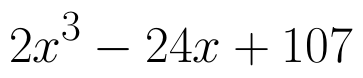the interval [1,3]. find the maximum value of the same function in [-3,-1]. (View Answer Video)

Question 3 : The normal to the curve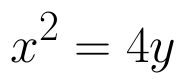passing (1, 2) is : (View Answer Video)

Question 4 : What is the maximum value of the function sin x+ cos x? (View Answer Video)

Question 5 : The slope of the normal to the curve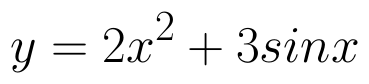at x = 0 is :

### Vector Algebra

Question 1 : Find the unit vector in the direction of vector PQ, where P and Q are the points (1, 2, 3) and (4, 5, 6) respectively. (View Answer Video)

Question 2 : Find the unit vector in the direction of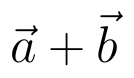if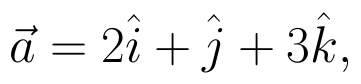and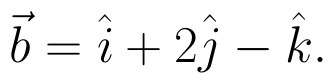(View Answer Video)

Question 3 : Find the position vector of a point which divides the join of points with position vectors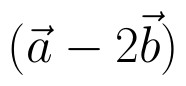and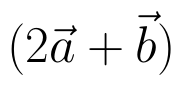externally in the ration 2:1.  (View Answer Video)

Question 4 :  Write the value of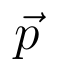for which the vectors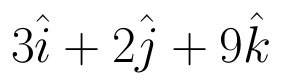and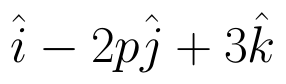are parallel vectors.  (View Answer Video)

Question 5 : Compute the magnitude of the following vectors: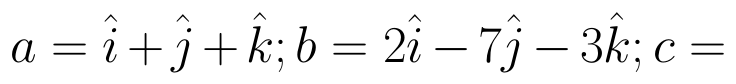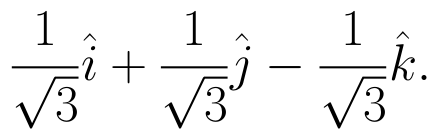(View Answer Video)

### Three Dimensional Geometry

Question 1 : If a line makes angle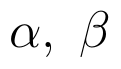and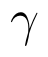with the coordinate axis, then find the value of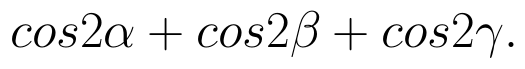(View Answer Video)

Question 2 : Find the angle between the lines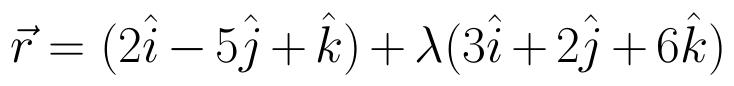and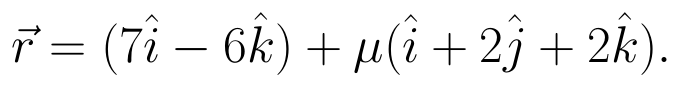(View Answer Video)

Question 3 : The equation of a line AB is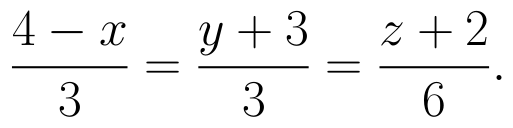Write the direction cosines of a line parallel to the above line. (View Answer Video)

Question 4 : If a line marks angles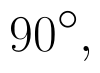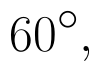and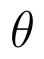with x, y and z-axis respectively, whereis acute, then find. (View Answer Video)

Question 5 : Find the equation of the plane passing through the line of intersection of the plane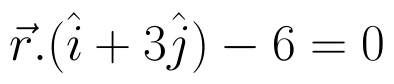and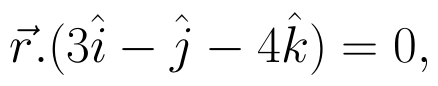which is at a unit distance from the origin. (View Answer Video)

### Application of Integrals

Question 1 : Using integration, find the area of the region bounded by the line 2x + y = 4, 3x - 2y = 6 and x - 3y + 5 = 0. (View Answer Video)

Question 2 : Using integration, find the area of the triangle ABC, where A is (2,3), B is (4,7) and C is (6,2). (View Answer Video)

Question 3 : Using the method of integration, find the area of the region bounded by the lines: 5x - 2y -10 = 0, x + y - 9 = 0 and 2x - 5y - 4 = 0. (View Answer Video)

Question 4 : Using integration, find the area of the region bounded by the triangle whose vertices are (-1, 2), (1, 5) and (3, 4). (View Answer Video)

Question 5 : Find the area of the region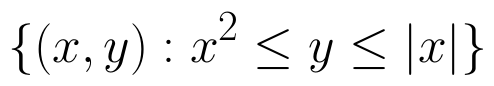. (View Answer Video)